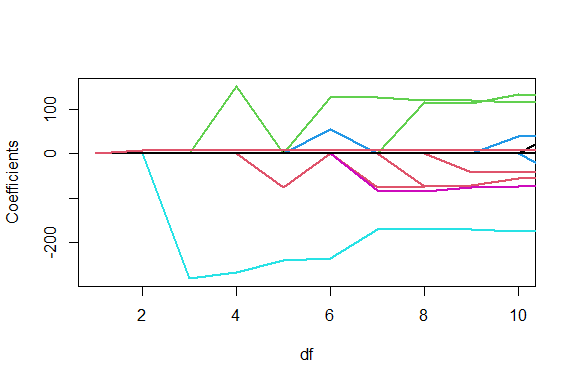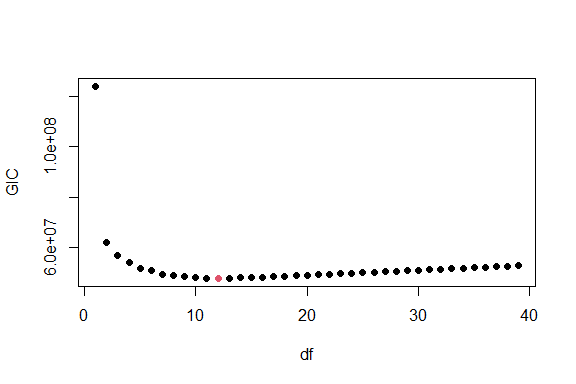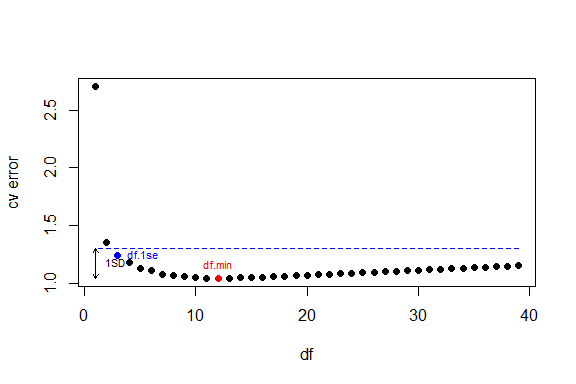# This document

The purpose of this vignette is to introduce readers to DMRnet package, bring them up to speed by providing a simple use case example and point interested readers towards more comprehensive informative material.

# DMRnet package

DMRnet is an R package for regression and classification with a family of model selection algorithms. DMRnet package supports both continuous and categorical predictors. The overall number of regressors may exceed the number of observations.

The selected model consists of a subset of numerical regressors and partitions of levels of factors.

The available model selection algorithms are the following:

• DMRnet is the default and the most comprehensive model selection algorithm in the package, it can be used both for $$p<n$$ and $$p \geq n$$ scenarios. It first executes a screening step in order to reduce the problem to $$p<n$$ and then uses DMR subsequently.
• GLAMER stands for Group LAsso MERger and it is a new (simplified in relation to DMRnet) model selection algorithm that can be used both for $$p<n$$ and $$p \geq n$$ scenarios. In comparison to DMRnet, the step of reordering variables based on their t-statistics is skipped. This is the first partition selection algorithm in literature for which a partition selection consistency has been proven for high dimensional scenario [Nowakowski et al., 2021].
• PDMR stands for Plain DMR and it is a threshold parameter agnostic variant of GLAMER algorithm [Nowakowski et al., 2023]. It can be used both for $$p<n$$ and $$p \geq n$$ scenarios, as well.
• Variable selection algorithm allows user to select (or deselect) full variables, for factors it means that merging their levels is not allowed (they are either retained or discarded in full). Internally, it is executed as a thresholded group lasso. Initially, a two dimensional net of lasso-related lambda and threshold values results in a large family of models, but at a later stage of the algorithm, for each model dimension the best model (i.e. the model maximizing the likelihood) is chosen.
• DMR [Maj-Kańska et al., 2015] is a model selection algorithm that can be used for $$p<n$$ scenario only.
• SOSnet [Pokarowski and Mielniczuk, 2015, Pokarowski et al., 2022] is a model selection algorithm that is used both for $$p<n$$ and $$p \geq n$$ scenarios for continuous-only regressors (with no categorical variables and, consequently, no partition selection).

Algorithm-wise, the package user has the following choices:

• The user can select DMRnet algorithm by calling DMRnet() or cv.DMRnet() functions.
• The user can select variable selection, GLAMER or PDMR algorithms by calling DMRnet() or cv.DMRnet() functions and passing algorithm="var_sel", algorithm="glamer" or algorithm="PDMR" argument, respectively.
• The user can select DMR algorithm by calling DMR() or cv.DMR() functions.
• The SOSnet algorithm is automatically chosen if DMRnet() or cv.DMRnet() functions were called with an input consisting of continuous-only regressors.

As this vignette is introductory only, and the choice of an algorithm is a somewhat more advanced topic, from now on it is assumed that the user works with DMRnet algorithm only.

# OK, so let’s get started

To load the package into memory execute the following line:

library(DMRnet)
#> To acknowledge our work please cite DMRnet in publications as:
#> Szymon Nowakowski, Piotr Pokarowski, Wojciech Rejchel, Agnieszka Sołtys, 2023. Improving Group Lasso for High-Dimensional Categorical Data. In: Computational Science – ICCS 2023. Lecture Notes in Computer Science, vol 14074, p. 455-470. Springer, Cham. doi:10.1007/978-3-031-36021-3_47

DMRnet package features two built-in data sets: Promoter and Miete [Fahrmeir et al., 2004].

We will work with Miete data set in this vignette. The Miete data consists of $$n = 2053$$ households interviewed for the Munich rent standard 2003. The response is continuous, it is monthly rent per square meter in Euros. There are 9 categorical and 2 continuous variables.

data("miete")
X <- miete[,-1]
y <- miete$rent head(X) #> bathextra tiles area kitchen rooms best good warm central year size #> 1 0 0 2 0 2 0 1 0 0 1918 68 #> 2 0 0 2 0 2 0 1 0 0 1995 65 #> 3 0 0 2 0 3 0 1 0 0 1918 63 #> 4 1 0 16 0 3 0 0 0 0 1983 65 #> 5 1 0 16 1 4 0 1 0 0 1995 100 #> 6 0 0 16 0 4 0 0 0 0 1980 81 Out of 9 categorical variables 7 have 2 levels each, and the two remaining (area and rooms) have 25 and 6 levels, respectively. This sums up to 45 levels. The way DMRnet handles levels results in 36 parameters for the categorical variables (the first level in each categorical variable is already considered included in the intercept). The 2 continuous variables give 2 additional parameters, the intercept is one more, so it totals in $$p = 39$$. # Estimating and inspecting a sequence of models In contrast to explicit group lasso methods which need a design matrix with explicit groups, DMRnet package internally transforms an input matrix into a design matrix (e.g. by expanding factors into a set of one-hot encoded dummy variables). Thus, X needs no additional changes and already is all set to be fed into DMRnet: models <- DMRnet(X, y, family="gaussian") models #> #> Arguments: #>$family
#>  "gaussian"
#>
#> $clust.method #>  "complete" #> #>$o
#>  5
#>
#> $nlambda #>  100 #> #>$maxp
#>  1027
#>
#> $lambda #> NULL #> #>$algorithm
#>  "DMRnet"
#>
#> Family of models:
#>  [1,] 39  44889691
#>  [2,] 38  44889694
#>  [3,] 37  44889702
#>  [4,] 36  44889714
#>  [5,] 35  44889736
#>  [6,] 34  44889761
#>  [7,] 33  44889800
#>  [8,] 32  44889868
#>  [9,] 31  44890015
#> [10,] 30  44890219
#> [11,] 29  44890407
#> [12,] 28  44890641
#> [13,] 27  44890887
#> [14,] 26  44891333
#> [15,] 25  44892150
#> [16,] 24  44892640
#> [17,] 23  44894059
#> [18,] 22  44898820
#> [19,] 21  44904071
#> [20,] 20  44910418
#> [21,] 19  44921810
#> [22,] 18  44930083
#> [23,] 17  44958900
#> [24,] 16  45063641
#> [25,] 15  45106343
#> [26,] 14  45232724
#> [27,] 13  45290696
#> [28,] 12  45367049
#> [29,] 11  45699667
#> [30,] 10  46235150
#> [31,]  9  46865054
#> [32,]  8  47378415
#> [33,]  7  48034125
#> [34,]  6  49694598
#> [35,]  5  50786340
#> [36,]  4  53188046
#> [37,]  3  56328078
#> [38,]  2  61742060
#> [39,]  1 123608575

Here, family="gaussian" argument was used to indicate, that we are interested in a linear regression for modeling a continuous response variable. The family="gaussian" is the default and could have been omitted as well. In a printout above you can notice a bunch of other default values set for some other parameters (e.g. nlambda=100 requesting the cardinality of a net of lambda values used) in model estimation.

The last part of a printout above presents a sequence of models estimated from X. Notice the models have varying number of parameters (model dimension df ranges from 39 for a full model down to 1 for the 39-th model).

Let us plot the path for the coefficients for the 10 smallest models as a function of their model dimension df:

plot(models, xlim=c(1, 10), lwd=2)Notice how you can also pass other parameters (xlim=c(1, 10), lwd=2) to change the default behavior of the plot() function.

To inspect the coefficients for a particular model in detail, one can use the coef() function. For the sake of the example, let’s examine the last model from the plot, with df=10:

coef(models, df=10)
#>  (Intercept)       tiles1        area7       area10       area11       area14
#> -2696.776884   -41.326719   -55.358136   -55.358136   -55.358136   -55.358136
#>       area16       area17       area19       area20       area22       area23
#>   -55.358136   -55.358136   -55.358136   -55.358136   -55.358136   -55.358136
#>       area24       area25     kitchen1        best1        good1        warm1
#>   -55.358136   -55.358136   115.522301   133.699574    39.478992  -173.480798
#>     central1         year         size
#>   -73.402473     1.428681     6.950459

Notice how area-related coefficients are all equal, effectively merging all 12 listed area levels with a coefficient -55.36 and merging all 13 remaining area levels with a coefficient 0.0. The 13 remaining area levels merged with a coefficient 0.0 were unlisted in a printout above. The reason behind it is that only non-zero coefficients get listed in the coef() function.

# Selecting best models

There are two basic methods for model selection supported in DMRnet package. One is based on a $$K$$-fold Cross Validation (CV), the other is based on Generalized Information Criterion (GIC) [Foster and George, 1994].

### Model selection with GIC

A GIC-based model selection is performed directly on a precomputed sequence of models

gic.model <- gic.DMR(models)
plot(gic.model)One can read a model dimension with

gic.model$df.min #>  12 One also has access to the best model coefficients with coef(gic.model) #> (Intercept) bathextra1 tiles1 area7 area10 area11 #> -2736.453989 59.260643 -42.372063 -55.249855 -55.249855 -55.249855 #> area14 area15 area16 area17 area19 area20 #> -55.249855 -55.249855 -55.249855 -55.249855 -55.249855 -55.249855 #> area21 area22 area23 area24 area25 kitchen1 #> -55.249855 -55.249855 -55.249855 -55.249855 -55.249855 111.371688 #> rooms4 rooms5 rooms6 best1 good1 warm1 #> -44.187398 -44.187398 -44.187398 127.381589 39.499137 -168.441539 #> central1 year size #> -74.219946 1.443990 7.156026 ### Model selection with $$K$$-fold Cross Validation The default $$K$$ value is 10. Because the data needs to be partitioned into CV subsets which alternate as training and validating sets, the precomputed sequence of models in models variable cannot be used directly, as was the case with GIC-based model selection. Instead, to perform a 10-fold CV-based model selection execute cv.model <- cv.DMRnet(X, y) plot(cv.model)As before, one can access the best model dimension with cv.model$df.min
#>  12

In a plot above there is also a blue dot indicating a df.1se model. This is the smallest model (respective to its dimension) having its error within the boundary of one standard deviation (the blue dotted line) from the best model. This model improves interpretability without erring much more than the best model.

cv.model$df.1se #>  3 Returning to the best model, df.min, its dimension is the same as when we used GIC directly. Let’s check if the CV-selected model is the same as the best model selected with GIC: coef(cv.model)==coef(gic.model) #> (Intercept) bathextra1 tiles1 area7 area10 area11 #> TRUE TRUE TRUE TRUE TRUE TRUE #> area14 area15 area16 area17 area19 area20 #> TRUE TRUE TRUE TRUE TRUE TRUE #> area21 area22 area23 area24 area25 kitchen1 #> TRUE TRUE TRUE TRUE TRUE TRUE #> rooms4 rooms5 rooms6 best1 good1 warm1 #> TRUE TRUE TRUE TRUE TRUE TRUE #> central1 year size #> TRUE TRUE TRUE # Predicting response for new observations Models selected with both model selection methods can be used to predict response variable values for new observations: predict(gic.model, newx=head(X)) #> [,1] #> 1 559.2277 #> 2 648.9469 #> 3 523.4476 #> 4 596.1306 #> 5 970.6029 #> 6 602.8470 predict(cv.model, newx=head(X)) #> [,1] #> 1 559.2277 #> 2 648.9469 #> 3 523.4476 #> 4 596.1306 #> 5 970.6029 #> 6 602.8470 No wonder the corresponding predictions are all equal, since the selected models were the same. In models selected with CV, one can switch between df.min and df.1se models with the use of md argument, as follows: predict(cv.model, md="df.min", newx=head(X)) # the default, best model #> [,1] #> 1 559.2277 #> 2 648.9469 #> 3 523.4476 #> 4 596.1306 #> 5 970.6029 #> 6 602.8470 predict(cv.model, md="df.1se", newx=head(X)) # the alternative df.1se model #> [,1] #> 1 568.6685 #> 2 547.5318 #> 3 533.4407 #> 4 547.5318 #> 5 794.1263 #> 6 660.2607 One can predict the response variable values for the whole sequence of models as well: predict(models, newx=head(X)) #> df39 df38 df37 df36 df35 df34 df33 df32 #> 1 570.1916 570.1880 570.1944 570.2044 570.2462 570.2468 570.2519 570.2554 #> 2 663.6774 663.6772 663.7068 663.7338 663.7017 663.7233 663.7777 663.8257 #> 3 519.4320 519.4302 519.4352 519.4451 519.4860 519.4831 519.4827 519.4941 #> 4 568.8714 568.8747 568.8634 568.8770 568.8583 568.8622 568.8667 568.8647 #> 5 948.5308 948.5381 948.5760 948.6384 948.6232 948.6464 948.6823 948.7183 #> 6 583.6860 583.6893 583.6874 583.6696 583.6517 583.6554 583.6507 583.6619 #> df31 df30 df29 df28 df27 df26 df25 df24 #> 1 570.2455 570.2503 569.4073 569.4309 569.4629 569.4797 569.5341 569.1385 #> 2 663.8759 663.8688 662.9924 662.9661 662.9836 662.9517 662.8686 662.3913 #> 3 519.4867 519.5139 518.6397 518.6799 518.6922 518.7602 518.7982 518.4336 #> 4 568.8863 568.8725 568.8900 568.8979 568.2476 568.2456 568.1406 568.1838 #> 5 948.7620 948.6792 948.5951 948.6107 947.9537 947.8719 947.6397 947.5754 #> 6 583.6725 583.6609 583.6405 583.6242 583.0231 583.0253 583.0193 582.9143 #> df23 df22 df21 df20 df19 df18 df17 df16 #> 1 569.0645 569.1271 569.4311 570.5289 570.8667 570.3454 570.3404 562.3298 #> 2 662.3284 662.6799 662.7128 663.9165 663.8429 663.4175 663.1364 652.6998 #> 3 518.4404 518.3905 518.6901 519.9875 520.3221 520.7543 521.0591 512.5275 #> 4 568.1961 568.3222 568.1244 569.0227 568.8951 569.5745 581.6448 580.8725 #> 5 947.3725 948.2670 948.6530 948.6425 949.1207 947.5811 959.4972 960.5421 #> 6 582.8929 582.9015 582.6029 583.3883 583.1541 581.7241 594.4963 592.5491 #> df15 df14 df13 df12 df11 df10 df9 df8 #> 1 556.3796 557.3476 557.1075 559.2277 553.6168 555.5438 537.3356 528.6948 #> 2 647.3305 647.7989 647.2196 648.9469 645.3276 644.7009 626.9365 622.1707 #> 3 520.5629 521.4951 521.4796 523.4476 519.7666 520.7916 502.3516 493.5944 #> 4 587.4935 597.0187 597.1792 596.1306 587.3871 532.7196 538.8990 531.8596 #> 5 961.7100 972.8877 970.1441 970.6029 997.1003 948.1311 919.9631 915.9963 #> 6 595.7414 604.5666 602.7236 602.8470 634.4291 639.6409 646.5391 639.7183 #> df7 df6 df5 df4 df3 df2 df1 #> 1 528.5285 533.6460 526.5034 557.4039 568.6685 559.0850 570.093 #> 2 621.0150 623.7535 650.1912 536.6915 547.5318 538.3833 570.093 #> 3 493.3071 498.1606 490.2621 522.8832 533.4407 524.5822 570.093 #> 4 527.6910 551.1119 552.7880 536.6915 547.5318 538.3833 570.093 #> 5 917.8128 997.3743 829.1419 929.4490 794.1263 779.9030 570.093 #> 6 635.9730 660.3250 663.0939 647.1579 660.2607 648.7923 570.093 Notice how the df12 model predictions overlap with the values we obtained for the df.min model, and df3 overlaps with the values we obtained for the df.1se model. # Classification with 2 classes For a classification task with 2 classes, the non-default family="binomial" should be provided in a call to DMRnet and cv.DMRnet (but not to gic.DMR) and the response variable should be a factor with two classes, with the last level in alphabetical order interpreted as the target class. It is illustrated with the following code with a somewhat artificial example: binomial_y <- factor(y > mean(y)) #changing Miete response var y into a binomial factor with 2 classes binomial_models <- DMRnet(X, binomial_y, family="binomial") gic.binomial_model <- gic.DMR(binomial_models) gic.binomial_model$df.min
#>  12

A corresponding predict call has a type parameter with the default value "link", which returns the linear predictors. To change that behavior one can substitute the default with type="response" to get the fitted probabilities or type="class" to get the class labels corresponding to the maximum probability. So to get actual values compatible with a binomial y, type="class" should be used:

predict(gic.binomial_model, newx=head(X), type="class")
#>   [,1]
#> 1    0
#> 2    1
#> 3    0
#> 4    1
#> 5    1
#> 6    1

Please note that 1 is the value of a target class in the predict output.